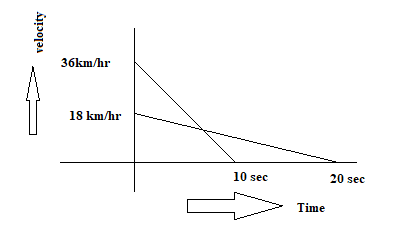﻿ Test Paper for Motion|Speed||Class 9 physics# Test Paper for Motion|Speed||Class 9 physics

Question 1.
What does the odometer of an automobiles measure? Under what condition is the magnitude of average velocity of an object equal to its average speed?

Question 2.
(a) What is the quantity which is measured by the area occupied below the velocity time graph?
(b) A train starting from rest attains a velocity of 72 km/h in 5 minutes. Assuming that the acceleration is uniform, find out the
(i) acceleration
(ii) the distance travelled by the train for attending this velocity?

Question 3
A bullet of mass 10g travelling horizontally with a velocity of 150m/s strikes a stationary wooden block  and comes to the rest in 0.03 s. calculate the distance of penetration of the bullet into the block. Also calculate the magnitude of the force exerted by the wooden block on the bullet.
Question 4.
Explain why is difficult for a fireman to hold a house, which ejects large amounts of water at a high velocity?
Question 5.
Differentiate between uniform acceleration and non uniform acceleration? Define one Newton.
Question 6.
A car is accelerated uniformly from 36 km per hour to 90 km per hour in 3 seconds. Calculate: (a) The acceleration of car   (b)The distance covered by the car in that time.
Question 7.
State Newton’s 1st law of motion? Why is it called as law of inertia? Define inertia? On what factors does inertia depends? Does inertia play any role for an object moving on a frictionless surface with uniform speed? Justify your answer. Which of the following has more inertia- 10kg cotton bale or 5kg iron block?

Question 8.
State Newon’s third law. Are the forces mentioned in the law balanced forces why? Will they always produce acceleration of equal magnitude? Why?
Question 9.
What is law of conservation of momentum? Derive it? Which would require a greater force to accelerate a ½ kg mass at 5 m/s2 or a 4 kg mass at 2 m/s2? Give reason.
Question 10.
What happens when we accidentally step on a peel of banana? Explain with reference to Newton’s law of motion.
Question 11.
Derive the velocity- position equation of motion (v2=u2+2aS) graphically (with the help of a velocity- time graph).
Question 12.
A motorcyclist riding motorcycle A who is travelling at 36 km/h applies the brakes and stops the motorcycle in 10 s. Another motorcyclist of motorcycle B who is travelling at 18 km/h applies the breaks and stops the motorcycle in 20s. Plot speed-time graph for the two motorcycles. Which of the two motorcycles traveled farther before it comes to a stop?
Question 13.
Abdul while driving to school, computes the average speed for his trip to be 20 km/h. on his return trio along th same route, there is less traffic and the average speed is 30km/h. what s the average speed for abdul’s trip?
Question 14.
A scooter is moving with an initial velocity of 90 km/hr and it takes 10s to stop it, after the brakes are applied. Calculate the force exerted by the brakes on the scooter if its mass along with the rider is 200 kg.What would be the force if the mass is halved?
Question 15.
(a) A body starts from rest and gains a velocity of 6 m/s in 2s of time. Find the acceleration of the body and its average velocity for the given time:
(b) A force of 24 N produces an acceleration of 3m/s2 on a body of mass m1 and an acceleration of 2m/s2 on a body of mass m2. What acceleration does it produce when applied on a combination of the two bodies?
1.
a. Odometer is a device that measures the distance travelled by an automobile based on the perimeter of the wheel as the wheel rotates. b. Average speed is equal to the magnitude of average velocity When an object moves along a straight line in the same direction. In this case its total path length is the magnitude of displacement.

2.
a. Displacement
b. v=72 km/hr =20 m/s , u=0 ,t=5 min=360 sec
$v=u+at$
a= .05 m/s2
$s=ut+\frac {1}{2}at^2$
s=3240 m

3. u=150m/s ,t=0.03 s, v=0 ,s=?
$v=u+at$
a=-5000 m/s2
$s=ut+\frac {1}{2}at^2$
s=2.25 m
Now
force = mass X acceleration
=.001 X 5000 =50 N

6. u=36 km/hr =10 m/s , v=90km/hr=25 m/s , t=3 sec , a=? ,s=?
$v=u+at$
a= 5m/s2
$s=ut+\frac {1}{2}at^2$
s=52.5 m

12.
Motorcycle A
u=36 km/hr =10 m/s , v=0 ,t=10 sec, s=?, a=?
$v=u+at$
a=-1m/s2
$s=ut+\frac {1}{2}at^2$
s=50 m

Motorcycle B
u=18 km/hr =5 m/s , v=0 ,t=20 sec, s=?, a=?
$v=u+at$
a=-.25 m/s2
$s=ut+\frac {1}{2}at^2$
s=50 m
Speed Time graph13. Let one way distance =x
Then time taken on one way with average speed 20 km/hr
$t_1=\frac {x}{20}$
Similarly time taken on return with average speed 30 km/hr
$t_2=\frac {x}{30}$

Total Time taken for the trip
$t = t_1 + t_2 = \frac {x}{20} + \frac {x}{30} =\frac {5x}{60}=\frac {x}{12}$

Total distance for the trip=2x
Average speed of the whole trip
$= \frac {Total \; distance \; for \; the \;trip}{Total \; Time \; taken \; for \; the \; trip}$
$= \frac {2x}{\frac {x}{12}} = 24 km/hr$

14.
u=90km/hr =25 m/s ,t=10 sec,a=?,v=0
$v=u+at$
a=-2.5 m/s2
Force applied(retarding)
$= mass \times acceleration$
$=200 \times 2.5 = 500 N$

If the mass is halved, force would be halved

15.
a. u=0, v=6 m/s ,t=2 sec, a=?
$v=u+at$
a= 3 m/s
Average velocity = 6/2 = 3m/s
b. Force applied= mass X acceleration
So for mass A
$24= 3 \times m_1$
$m_1=8kg$
So for mass B
$24= 2 \times m_2$
$m_2=12kg$
Combination of both the bodies
=8+12=20 Kg
Now
a= F/m = 24/20 =1.2 m/s2

Latest Articles
Synthetic Fibres and Plastics Class 8 Practice questions

Class 8 science chapter 5 extra questions and Answers

Mass Calculator

3 Fraction calculator

Garbage in Garbage out Extra Questions7